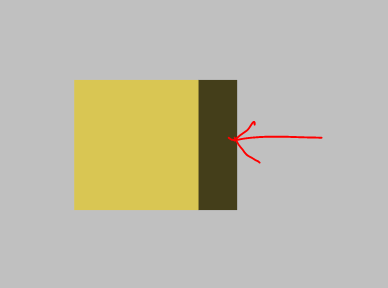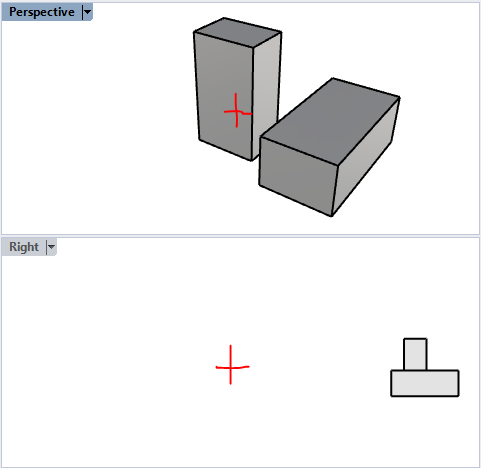# Parallel Projection not working

Hey All,

I am trying to make the ParallelProjection work and I am able to create a camera that uses parallel projection bu the I observe that it does not generate flat views that I expect. Following is what I am doing to generate that generates the attached image of a cube.``````import vtk

colors = vtk.vtkNamedColors()

# Create a rendering window and renderer.
ren = vtk.vtkRenderer()
renWin = vtk.vtkRenderWindow()
renWin.SetWindowName('Cube1')

# Create a renderwindowinteractor.
iren = vtk.vtkRenderWindowInteractor()
iren.SetRenderWindow(renWin)

# Create cube.
cube = vtk.vtkCubeSource()
cube.Update()

# mapper
cubeMapper = vtk.vtkPolyDataMapper()
cubeMapper.SetInputData(cube.GetOutput())

# Actor.
cubeActor = vtk.vtkActor()
cubeActor.SetMapper(cubeMapper)
cubeActor.GetProperty().SetColor(colors.GetColor3d('Banana'))

# Assign actor to the renderer.
ren.SetBackground(colors.GetColor3d('Silver'))

# Create a parallel projection camera
camera = vtk.vtkCamera()
camera.SetPosition(5,0,15)
camera.SetFocalPoint(0,0,-1)
camera.SetParallelProjection(True)
camera.ParallelProjectionOn()

ren.SetActiveCamera(camera)

# Enable user interface interactor.
iren.Initialize()
renWin.Render()
iren.Start()
``````

What am I doing wrong here?

I noticed that if I set the position to (0,0,15) then the side of the cube won’t be visible. But it shouldn’t be that way. I think parallel projection will work for any postion and I am doing something wrong here.

What I have shared is a sample. I am trying to make this work on scene with multiple actors such as the following image where I am trying to export the side view of a few buildings.

Looks correct to me. Your camera is offset by 5 in the x direction, so you should get an oblique view. If you change the camera position to (0,0,15) you will be looking directly at one side and see a square. The oblique side appears dark because vtk only uses one light by default - you can add the vtkLightKit to get lighting like Paraview.

1 Like

Thanks for commentng @Aron_Helser. I tried that earlier and it did work. Are you saying that that is how VTK works? I was expecting it to work without without doing that.

What if the actor is near (0,0,0) and you want to look at the actor from a distance, say (-15,0,2)?

Hello,

Perhaps the name VTK uses is a bit misleading. Parallel projection is actually a family of projections. The parallel projection we see in VTK is of type trimetric. There are other types of parallel projections such as cavalier, cabinet, isometric, etc. Perhaps the cabinet projection is what you’re looking for judging from your figure of buildings.

Perspective projections is also a family of projections: one-point projection, two-point projection, etc. The one called “perspective projection” in VTK is more accurately described as a three-point projection.

regards,

Paulo

2 Likes

Good to know @Paulo_Carvalho. Thanks for sharing

The following image shows two views of the same geometry. See the view named “right.” I am expecting such flat projection. Also observe that camera is point towards the center of the view not the buildings. This is the effect I am looking for in VTK. I have also tird orthogonalizeViewUp(), but that didn’t work either.``````vtkTransform transform = vtkTransform.New();
transform.RotateWXYZ(-90, 1, 0, 0);
camera.ApplyTransform(transform);``````

In general you need to have your camera position and camera focal point going along an axis, otherwise you will get an oblique view.

``````+ focalPoint

[Object]

+ Camera position
``````

vs

``````
+ focal point
[Object]
+ camera position
``````

I hope my ASCII art is making sense1 Like

Hello,

If I understood right, to achieve the effect you want, you could do this (it’s in C++ but it is not difficult to translate to Python):

``````void lookAtXY()
{
double *fp = m_renderer->GetActiveCamera()->GetFocalPoint();
double *p  = m_renderer->GetActiveCamera()->GetPosition();
double dist
= std::sqrt(  (p - fp) * (p - fp) + (p - fp) * (p - fp)
+ (p - fp) * (p - fp));
m_renderer->GetActiveCamera()->SetPosition(fp, fp, fp + dist);
m_renderer->GetActiveCamera()->SetViewUp(0.0, 1.0, 0.0);
// redraw the scene
m_renderWindow->Render();
}

void lookAtXZ()
{
double *fp = m_renderer->GetActiveCamera()->GetFocalPoint();
double *p  = m_renderer->GetActiveCamera()->GetPosition();
double dist
= std::sqrt(  (p - fp) * (p - fp) + (p - fp) * (p - fp)
+ (p - fp) * (p - fp));
m_renderer->GetActiveCamera()->SetPosition(fp, fp - dist, fp);
m_renderer->GetActiveCamera()->SetViewUp(0.0, 0.0, 1.0);
// redraw the scene
m_renderWindow->Render();
}

void lookAtYZ()
{
double *fp = m_renderer->GetActiveCamera()->GetFocalPoint();
double *p  = m_renderer->GetActiveCamera()->GetPosition();
double dist
= std::sqrt(  (p - fp) * (p - fp) + (p - fp) * (p - fp)
+ (p - fp) * (p - fp));
m_renderer->GetActiveCamera()->SetPosition(fp + dist, fp, fp);
m_renderer->GetActiveCamera()->SetViewUp(0.0, 0.0, 1.0);
// redraw the scene
m_renderWindow->Render();
}

(...)

lookAtXY(); //or lookAtXZ() or lookAtYZ() depending on how the objects are aligned in your scene
m_renderer->GetActiveCamera()->ParallelProjectionOn();

(...)
``````

Complete code here: gammaray/view3dwidget.cpp at master · PauloCarvalhoRJ/gammaray · GitHub .

I hope this helps,

Paulo

Thank you all for help. Appreciate it!